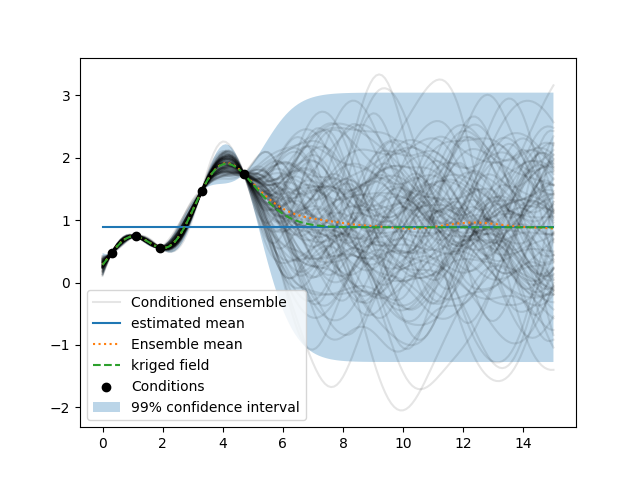# Example: Conditioning with Ordinary Kriging¶

Here we use ordinary kriging in 1D (for plotting reasons) with 5 given observations/conditions, to generate an ensemble of conditioned random fields. The estimated mean can be accessed by `srf.mean`.

```import numpy as np
import matplotlib.pyplot as plt
import gstools as gs

# condtions
cond_pos = [0.3, 1.9, 1.1, 3.3, 4.7]
cond_val = [0.47, 0.56, 0.74, 1.47, 1.74]
gridx = np.linspace(0.0, 15.0, 151)
```
```# spatial random field class
model = gs.Gaussian(dim=1, var=0.5, len_scale=2)
srf = gs.SRF(model)
srf.set_condition(cond_pos, cond_val, "ordinary")
```
```fields = []
for i in range(100):
# print(i) if i % 10 == 0 else None
fields.append(srf(gridx, seed=i))
label = "Conditioned ensemble" if i == 0 else None
plt.plot(gridx, fields[i], color="k", alpha=0.1, label=label)
plt.plot(gridx, np.full_like(gridx, srf.mean), label="estimated mean")
plt.plot(gridx, np.mean(fields, axis=0), linestyle=":", label="Ensemble mean")
plt.plot(gridx, srf.krige_field, linestyle="dashed", label="kriged field")
plt.scatter(cond_pos, cond_val, color="k", zorder=10, label="Conditions")
plt.legend()
plt.show()
```As you can see, the kriging field coincides with the ensemble mean of the conditioned random fields and the estimated mean is the mean of the far-field.

Total running time of the script: ( 0 minutes 1.799 seconds)

Gallery generated by Sphinx-Gallery